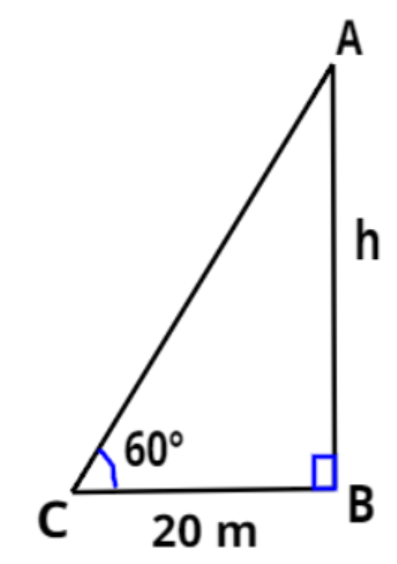QuestionAnswers

# A tower stands vertically on the ground. From a point on the ground, 20 m away from the foot of the tower, the angle of elevation of the top of the tower is ${60^0}$. What is the height of the tower?Verified
149.1k+ views
Hint- Here, we will be using the tangent of the angle of elevation in order to find the height of the tower.

Let AB is the tower of height $h$ meters and C be any point on the ground which is 20 m away from the foot of the tower and the angle of elevation of the top of the tower from point C is ${60^0}$.
Clearly, $\vartriangle {\text{ABC}}$ is a right angled triangle at vertex B.
As we know that in any right angled triangle, $\tan \theta = \dfrac{{{\text{Perpendicular}}}}{{{\text{Base}}}}$
From the figure we can say that according to angle ${60^0}$, AB is the perpendicular, BC is the base and
AC is the hypotenuse of the right angled triangle ABC.
So, $\tan {60^0} = \dfrac{{{\text{AB}}}}{{{\text{BC}}}} = \dfrac{h}{{20}} \Rightarrow h = 20\left( {\tan {{60}^0}} \right){\text{ }} \to {\text{(1)}}$
Also we know that $\tan {60^0} = \sqrt 3$
Equation (1) becomes $\Rightarrow h = 20\left( {\tan {{60}^0}} \right) = 20\sqrt 3 {\text{ m}}$
Hence, the height of the tower AB is $20\sqrt 3$ meters.

Note- In these type of problems we need to know that for any right angled triangle, the side opposite to
the right angle is the hypotenuse, the side opposite to the acute angle considered (in the above problem
the acute angle considered is ${60^0}$) is the perpendicular and the remaining side is the base.